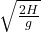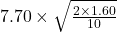## (URGENT) A ball rolls off a ledge. Its velocity is 7.70m/s in a horizontal direction. It falls on the floor 1.60m below the ledge. What is t

Question

(URGENT) A ball rolls off a ledge. Its velocity is 7.70m/s in a horizontal direction. It falls on the floor 1.60m below the ledge. What is the horizontal distance that it travels while in the air?

in progress 0
2 months 2021-07-24T20:57:33+00:00 1 Answers 2 views 0

the horizontal distance is 4.355 meters

Explanation:

The computation of the horizontal distance while travelling in the air is shown below:

Data provided in the question is as follows

Velocity = u = 7.70 m/s

H = 1.60 m

R = horizontal direction

Based on the above information

As we know that

R = u × time

where,

Time =So,

== 4.355 meters

hence, the horizontal distance is 4.355 meters